PSAT Math : Arithmetic

Example Questions

Example Question #71 : Arithmetic

A factory makes six widgets in a batch that takes 24 minutes to complete. How long does it take to make 57 widgets?

3 hours, 30 minutes

3 hours

3 hours, 5 minutes

3 hours, 45 minutes

4 hours

4 hours

Explanation:

We have here a basic ratio:

6/24 = 57/x

Solving for x, we get: 6x = 24 * 57; 6x = 1368; x = 228

Now, we must be very careful here, however. Is 228 an even multiple of 24?  228/24 = 9.5.  That means that we would have to do 9.5 batches.  The question indicates that these are batched in groups of 6; therefore, we have to run 10 batches to get our amount. That would be 240 minutes or 4 hours.

Example Question #72 : Arithmetic

Kendall can run 26 miles in 4 hours and 20 minutes. At this rate how long would it take him to run 100 miles?

12 hours and 20 minutes

15 hours and 50 minutes

10 hours and 55 minutes

16 hours and 40 minutes

18 hours and 35 minutes

16 hours and 40 minutes

Explanation:

Set up a proportion and conver 4 hours and 20 minutes into just minutes for now,

so 26/260 = 100/X

Solve for X = 1000 minutes

1000/60 = 16 hours and 40 minutes

Example Question #81 : Proportion / Ratio / Rate

A recipe calls for a ratio of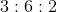for wheat, barley, and flour. If we have 12 pounds of barley, how much wheat do we need to use all of it?Explanation:

We can set up a ratio of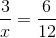and then solve forExample Question #82 : Proportion / Ratio / Rate

To ship a package, the postal service charges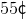for the first 150 grams and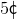for each additional 50 grams or part thereof. What is one possible weight in grams for a package that costs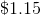to ship?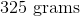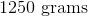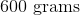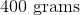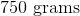Explanation:

The weight will be 150 grams + (1.15 – 0.55)/0.05 * 50g.

You need to subtract first 150 grams cost from the total cost and divide by the price per unit to determine how many units. Next, multiply units by weight per unit and add to the original first 150 grams.

150 + 600 = 750 grams

This is the maximum weight that can be sent at that price; the minimum weight that could be charged this price would be 701 grams. Hence a package weighing 750 grams will be charged \$1.15.

Example Question #83 : Proportion / Ratio / Rate

Six is to thirteen-and-a-half as seventeen is to what?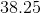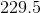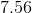None of the other answers are correct.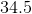Explanation:

Let us express this as an algebraic expression: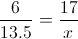We can cross multiply: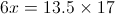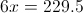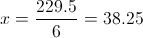Example Question #87 : Proportion / Ratio / Rate

Out of 85 students in a certain class, 42 own a laptop and 54 own an mp3 player. If 5 students don't own either, what fraction of the students own both a laptop and an mp3 player?

7/40

19/80

16/85

1/8

1/10

16/85

Explanation:

Once you subtract the 5 students that don't own either, there are 80 students left.

There's 96 total students when you add the number that own an mp3 and the number that own a laptop, meaning 16 own both.

Recall that the fraction will be number of students who have both laptop and mp3 divided by the total students in the class.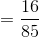Example Question #1 : How To Express A Fraction As A Ratio

express 7/8 as a ratio

not possible to express as a ratio

0.875

1.15

7:8

8:7

7:8

Explanation:

a ratio that comes from a fraction is the numerator: denominator

7/8 = 7:8

Example Question #2 : How To Express A Fraction As A Ratio

1 meter contains 100 centimeters.

Find the ratio of 1 meter and 40 centimeters to 1 meter:

12:5

2:3

7:5

9:6

3:2

7:5

Explanation:

1m 40cm = 140cm. 1m = 100cm. So the ratio is 140cm:100cm. This can be put as a fraction 140/100 and then reduced to 14/10 and further to 7/5. This, in turn, can be rewritten as a ratio as 7:5.

Example Question #34 : Proportion / Ratio / Rate

When television remotes are shipped from a certain factory, 1 out of every 200 is defective. What is the ratio of defective to nondefective remotes?

1:199

199:1

1:200

200:1

1:199

Explanation:

One remote is defective for every 199 non-defective remotes.

Example Question #35 : Proportion / Ratio / Rate

On a desk, there arepapers for everypaper clips andpapers for everygreeting card. What is the ratio of paper clips to total items on the desk?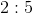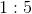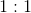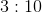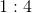Explanation:

Begin by making your life easier: presume that there arepapers on the desk. Immediately, we know that there arepaper clips. Now, if there arepapers, you know that there also must begreeting cards. Technically you figure this out by using the ratio: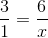By cross-multiplying you get: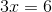Solving for, you clearly get.

(Many students will likely see this fact without doing the algebra, however. The numbers are rather simple.)

Now, this means that our desk has on it:paperspaper clipsgreeting cards

Therefore, you havetotal items.  Based on this, your ratio of paper clips to total items is: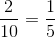, which is the same as.